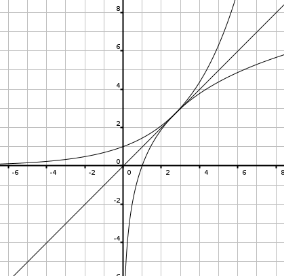Solution: Let $$f(x) = b^x$$ and $$g(x) = \log_b x$$. Because $$f$$ and $$g$$ are inverse functions, they are reflections of each other over the line $$y=x$$. Also, anywhere $$f$$ intersects $$g$$ will be at a point on $$y=x$$, including the point of tangency. Let $$(c,c)$$ be this point of tangency so that $$f(c) = c = g(c)$$.Because the tangent line to each of these functions at the point $$(c,c)$$ has a slope of 1, we can get useful information out of their derivatives. $$f'(x) = b^x \ln b$$ $$f'(c) = b^c \ln b = 1$$ $$g'(x) = \dfrac{1}{x \ln b}$$ $$g'(c) = \dfrac{1}{c \ln b} = 1$$ From $$g'(c)$$ we have: $$c \ln b = 1$$ $$c = \dfrac{1}{\ln b}$$ Then from $$f'(c)$$: $$f'(c) = b^{1/ \ln b} \ln b = 1$$ $$\ln(b^{1/ \ln b} \ln b) = \ln 1$$ $$\ln(b^{1/ \ln b}) + \ln(\ln b) = 0$$ $$(1 / \ln b) \ln b + \ln(\ln b) = 0$$ $$1 + \ln \ln b = 0$$ $$\ln \ln b = -1$$ $$\ln b = e^{-1} = 1/e$$ $$b = e^{1/e}$$ Therefore the base b is equal to $$e^{1/e}$$.